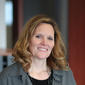# MathematicsSecondary Math Instructional SupervisorElementary STEM Instructional Supervisor

Our goal is to develop mathematically proficient students through a focus on reasoning and deeper understanding to prepare all students to succeed in a changing world. Mathematically proficient students communicate verbally, numerically, symbolically, and graphically by engaging in the Standards for Mathematical Practice.

These standards include: making sense of problems and persevering in solving them; reasoning abstractly and quantitatively; constructing viable arguments and critiquing the reasoning of others; modeling with mathematics; using appropriate tools strategically; attending to precision; looking for and making use of structure; and looking for and expressing regularity in repeated reasoning.

Students in Grade 6 Math will work to connect ratio and rate to whole-number multiplication and division and use concepts of ratio and rate to solve problems; understand division of fractions and extend the notion of number to the system of rational numbers, including negative numbers; write, interpret and use expressions and equations; and develop understanding of statistical thinking.

Units of Study include:

• Use Positive Rational Numbers
• Integers and Rational Numbers
• Numeric and Algebraic Expressions
• Represent and Solve Equations and Inequalities
• Understand and Use Ratio and Rate
• Understand and Use Percent
• Solve Area, Surface Area and Volume
• Display, Describe and Summarize Data

Students in Grade 7 Math will develop understanding of and apply proportional relationships; develop understanding of operations with rational numbers and work with expressions and linear equations; solve problems involving scale drawings and informal geometric constructions, and work with two and three dimensional shapes to solve problems involving area, surface area, and volume; and draw inferences about populations based on samples. Grade 7 Honors Math will cover all of the topics covered in Grade 7 Math and embed standards from grade 8 to prepare students that may accelerate to Algebra I.

Units of Study include:

• Integers and Rational Numbers
• Analyze and Use Proportional Relationships
• Analyze and Solve Percent Problems
• Generate Equivalent Expressions
• Solve Problems Using Equations and Inequalities
• Use Sampling to Draw Inferences about Populations
• Probability
• Geometry – Circles, Angle Relationships and Solids

Students in Grade 8 Math will formulate and reason about expressions and equations; grasp the concept of a function and use functions to describe quantitative relationships; analyze two and three dimensional space and figures using distance, angle, similarity and congruence; and understand and apply the Pythagorean Theorem.

Units of Study include:

• Real Numbers
• Analyze and Solve Linear Equations
• Analyze and Solve Systems of Linear Equations
• Use Functions to Model Relationships
• Investigate Bivariate Data
• Congruence and Similarity

High school Mathematics classes include the following. For a full list of what's offered at Albany High, please review our course offerings book.

### Algebra 1

Algebra 1 is the first Regents mathematics course for high school students and ends with the Algebra Regents exam in June. This course will broaden mathematical knowledge while developing algebraic skills and processes that allow the student to use a variety of techniques to solve problems in a variety of settings.

### Geometry R (Regents Level)

Geometry is the second course of the math Regents sequence for high school students who have passed Algebra Regents. Topics covered will include congruence, similarity, trigonometry, volume, proofs, coordinate geometry and circles.

### Algebra 2/IB Mathematics SL 1

Algebra 2/IB Mathematics SL 1 is the third course of the Regents math sequence leading to a Regents examination and the first year of a two-year IB sequence in standard level mathematics assessed in the second year. The course includes an extension of the study of functions that started in Algebra 1. It will include rational expressions, exponential and logarithmic functions, the complex number system, fundamentals of trigonometric functions and a basic study of probability and statistics.